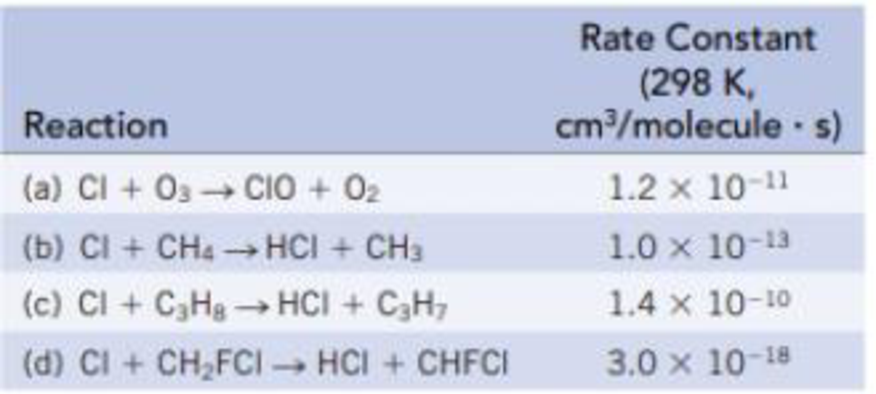Chapter 14, Problem 72GQ

Chapter
Section
Textbook Problem

Hundreds of different reactions occur in the stratosphere, among them reactions that destroy the Earth’s ozone layer. The table below lists several (second-order) reactions of Cl atoms with ozone and organic compounds; each is given with its rate constant.For equal concentrations of Cl and the other reactant, which is the slowest reaction? Which is the fastest reaction?

Interpretation Introduction

Interpretation:

From the given set of reactions, the fastest reaction and the slowest reaction should be identified.

Concept introduction:

Rate law or rate equation: Rate law:

It is generally the rate equation that consists of the reaction rate with the concentration or the pressures of the reactants and constant parameters.

aA + bBxXRate of reaction = k [A]m[B]n

Order of a reaction:  The order of each reactant in a reaction is represented by the exponential term of the respective reactant present in the rate law and the overall order of the reaction is the sum of all the exponents of all reactants present in the chemical reaction. The order of the reaction is directly proportional to the concentration of the reactants.

Rate constant, k: The rate constant for a chemical reaction is the proportionality term in the chemical reaction rate law which gives the relationship between the rate and the concentration of the reactant present in the chemical reaction.

Explanation

Reason for correct answer:

Examining the given table it is clear that Reaction(d) is the slowest and Reaction(c) is the fastest reaction since reaction rate is based on the rate constant value.

The higher the rate constant value faster the reaction will be as Rate=kdAdtthatisRat

Still sussing out bartleby?

Check out a sample textbook solution.

See a sample solution

The Solution to Your Study Problems

Bartleby provides explanations to thousands of textbook problems written by our experts, many with advanced degrees!

Get Started